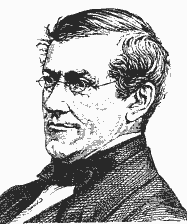Home » » Wheatstone Bridge and Wheatstone Bridge Theory

# Wheatstone Bridge

For measuring accurately any resistance Wheatstone Bridge is widely used. There are two known resistors, one variable resistor and one unknown resistor connected in bridge form as shown below. By adjusting the variable resistor the current through the Galvanometer is made zero. When the current through the galvanometer becomes zero, the ratio of two known resistors is exactly equal to the ratio of adjusted value of variable resistance and the value of unknown resistance. In this way the value of unknown resistance can easily be measured by using a Wheatstone Bridge.

## Wheatstone Bridge Theory

The general arrangement of Wheatstone bridge circuitis shown in the figure below. It is a four arms bridge circuit where arm AB, BC, CD and AD are consisting of resistances P, Q, S and R respectively. Among these resistances P and Q are known fixed resistances and these two arms are referred as ratio arms. An accurate and sensitive Galvanometer is connected between the terminals B and D through a switch S2. The voltage source of this Wheatstone bridge is connected to the terminals A and C via a switch S1 as shown. A variable resistor S is connected between point C and D. The potential at point D can be varied by adjusting the value of variable resistor. Suppose current I1 and current I2 are flowing through the paths ABC and ADC respectively. If we vary the resistance value of arm CD the value of current I2 will also be varied as the voltage across A and C is fixed. If we continue to adjust the variable resistance one situation may comes when voltage drop across the resistor S that is I2.S is becomes exactly equal to voltage drop across resistor Q that is I1.Q. Thus the potential at point B becomes equal to the potential at point D hence potential difference between these two points is zero hence current through galvanometer is nil. Then the deflection in the galvanometer is nil when the switch S2 is closed.

Now, from Wheatstone bridge circuit

 current I1 = V P + Q

and

 current I2 = V R + S

Now potential of point B in respect of point C is nothing but the voltage drop across the resistor Q and this is

 I1.Q = V.Q -------------------(i) P + Q

Again potential of point D in respect of point C is nothing but the voltage drop across the resistor S and this is

 I2.S = V.S ------------------(ii) R + SEquating, equations (i) and (ii) we get,

 V.Q = V.S ⇒ Q = S P + Q R + S P + Q R + S
 ⇒ P + Q = R + S ⇒ P + 1 = R + 1 ⇒ P = R Q S Q S Q S
 ⇒ R = SX P Q

Here in the above equation, the value of S and P ⁄ Q are known, so value of R can easily be determined.

The resistances P and Q of the Wheatstone bridge are made of definite raio such as 1:1; 10:1 or 100:1 known as ratio arms and S the reostat arm is made continuously variable from 1 to 1,000 Ω or from 1 to 10,000 Ω

The above explanation is most basic Wheatstone Bridge theory.

--